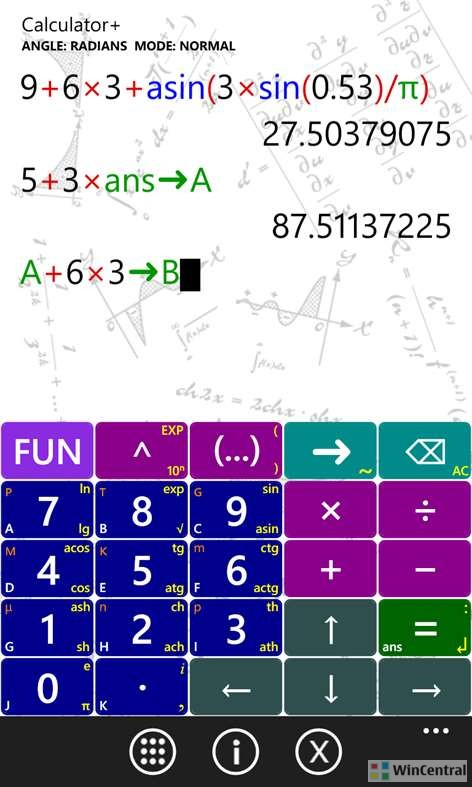The Windows Phone app which has gone free next as myAppfree app of the day is Calculator+. Thi  is a great replacement for your existing Windows Phone calculator.

This app allows ordinary and scientific calculations. Calculator+ is the most functional calculator in the Windows phone store!.

### Calculator+ Features:

– plots of function

– complex numbers

– matrices

– bin, oct, hex calculation modes

– 26 variables, 26 matrix variables, 26 list variables

– math constants

– mathematical, trigonometrical, hyperbolic, special functions

– History of calculations

– dark and light themes

– equation solver (SLE and polynomical)

– CAS GIAC module (symbolic computations like integration, simplification, differentiation and e.t.c)

– Ability to add own functions